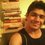# Principle of Mathematical Induction?

$\large (\ \underbrace{ 66666\ldots6 }_{n \text{ number of 6's}} \ )^2+ \underbrace{88888\ldots8}_{n \text{ number of 8's}}= \underbrace{44444\ldots4}_{2n \text{ number of 4's}}$

Prove the above equation of positive integer $n$.

Go through more proofs via Proofs - Rigorous Mathematics and enhance your mathematical growth!Note by Sandeep Bhardwaj
5 years, 10 months ago

This discussion board is a place to discuss our Daily Challenges and the math and science related to those challenges. Explanations are more than just a solution — they should explain the steps and thinking strategies that you used to obtain the solution. Comments should further the discussion of math and science.

When posting on Brilliant:

• Use the emojis to react to an explanation, whether you're congratulating a job well done , or just really confused .
• Ask specific questions about the challenge or the steps in somebody's explanation. Well-posed questions can add a lot to the discussion, but posting "I don't understand!" doesn't help anyone.
• Try to contribute something new to the discussion, whether it is an extension, generalization or other idea related to the challenge.
• Stay on topic — we're all here to learn more about math and science, not to hear about your favorite get-rich-quick scheme or current world events.

MarkdownAppears as
*italics* or _italics_ italics
**bold** or __bold__ bold
- bulleted- list
• bulleted
• list
1. numbered2. list
1. numbered
2. list
Note: you must add a full line of space before and after lists for them to show up correctly
paragraph 1paragraph 2

paragraph 1

paragraph 2

[example link](https://brilliant.org)example link
> This is a quote
This is a quote
    # I indented these lines
# 4 spaces, and now they show
# up as a code block.

print "hello world"
# I indented these lines
# 4 spaces, and now they show
# up as a code block.

print "hello world"
MathAppears as
Remember to wrap math in $$ ... $$ or $ ... $ to ensure proper formatting.
2 \times 3 $2 \times 3$
2^{34} $2^{34}$
a_{i-1} $a_{i-1}$
\frac{2}{3} $\frac{2}{3}$
\sqrt{2} $\sqrt{2}$
\sum_{i=1}^3 $\sum_{i=1}^3$
\sin \theta $\sin \theta$
\boxed{123} $\boxed{123}$

## Comments

Sort by:

Top Newest

$\underbrace{ 6666\ldots6 }_{n \text{ numbers of 6's}} = \dfrac{6}{9}(10^n - 1) = \dfrac{2}{3}(10^n - 1)$ $\underbrace{8888\ldots8 }_{n \text{ numbers of 8's}} = \dfrac{8}{9}(10^n - 1)$ $\underbrace{ 4444\ldots4 }_{2n \text{ numbers of 4's}} = \dfrac{4}{9}(10^{2n} - 1)$

So, $(\underbrace{ 6666\ldots6 }_{n \text{ numbers of 6's}})^2 + \underbrace{8888 \ldots8}_{n \text{number of 8's}} = (\dfrac{2}{3}(10^n - 1))^{2} + \dfrac{8}{9}(10^n - 1)$ $=\dfrac{4}{9}(10^{2n} - 2 \times 10^n +1 + 2 \times 10^n -2)$ $=\dfrac{4}{9}(10^{2n} - 1)$ $=\underbrace{4444 \ldots 4}_{2n \text{ number of 4's}}$

Hence, proved.

- 5 years, 10 months ago

Log in to reply

Great! Well done!

- 5 years, 10 months ago

Log in to reply

Thank u

- 5 years, 10 months ago

Log in to reply

For the sake of the title of this note, can you prove this using mathematical induction?

- 5 years, 10 months ago

Log in to reply

Hi @Pi Han Goh , I have even posted the solution using Induction. By the way can u please add me as your friend in Facebook. It's request from me.

- 5 years, 10 months ago

Log in to reply

Observe that $6^2 + 8 = 44$. So, it is true for $n=1$.

Let us suppose that for some $k$,

$(\underbrace{ 6666\ldots6 }_{k \text{ numbers of 6's}})^{2} + \underbrace{8888\ldots8 }_{k \text{ numbers of 8's}} = \underbrace{ 4444\ldots4 }_{2k \text{ numbers of 4's}}$

So, Consider

$(\underbrace{ 6666\ldots6 }_{k+1 \text{ numbers of 6's}})^{2} + \underbrace{8888\ldots8 }_{k+1 \text{ numbers of 8's}}$ $=(6 \times 10^{k} +\underbrace{ 6666\ldots6 }_{k \text{ numbers of 6's}})^{2} + 8 \times 10^{k} +\underbrace{8888\ldots8 }_{k \text{ numbers of 8's}}$ $=36 \times 10^{2k} + 2 \times 6 \times 10^{k} \times \underbrace{ 6666\ldots6 }_{k \text{ numbers of 6's}} +(\underbrace{ 6666\ldots6 }_{k \text{ numbers of 6's}})^{2} + 8 \times 10^{k} + \underbrace{8888\ldots8 }_{k \text{ numbers of 8's}}$ $= 36 \times 10^{2k} + 2 \times 6 \times 10^{k} \times \underbrace{ 6666\ldots6 }_{k \text{ numbers of 6's}} + 8 \times 10^{k} +\underbrace{ 4444\ldots4 }_{2k \text{ numbers of 4's}}$ $= 36 \times 10^{2k} + 2 \times 6 \times 10^{k} \times \dfrac{6}{9}(10^{k} - 1) + 8 \times 10^{k} +\underbrace{ 4444\ldots4 }_{2k \text{ numbers of 4's}}$ $= 44 \times 10^{2k} + \underbrace{ 4444\ldots4 }_{2k \text{ numbers of 4's}}$ $= \underbrace{ 4444\ldots4 }_{2k+2 \text{ numbers of 4's}}$

So, by $\text{Principle of Finite Mathematical Induction}$. The given statement is true.

- 5 years, 10 months ago

Log in to reply

×

Problem Loading...

Note Loading...

Set Loading...